Welcome to Study Material Solution
Leave Comment

# MAGNETISM

Magnet was first discovered some 5,000 years ago in Magnesia. It could attract small pieces of iron towards it and had directional property i.e. when suspended freely, it always points in north-south direction. There is a true story behind its discovery.

(A) Discovery of Magnet: There was a shepherd boy named Magnaus in the town Magnesia in Greece. He had wooden stuff attached to iron sole. One day he left it in a mine. When he came back, the iron sole was firmly attacked to the roof. He got terrified and thought it to be work of some evil spirit or ghost. The roof was actually an iron ore (Fe3O4) magnetite. This first discovered magnet was called magnetite or natural magnet. It points in a particular (N–S) direction. It is also called loading stone which has now been changed to lode stone.
Gilbert made detailed study of magnets and their properties. Magnets are essential parts of all generators used for the production of electricity. They also form essential parts of electric motors, TV., radio, stereos and large number of instruments.

(B)  Properties of Magnets
(i) It attracts small pieces of iron towards it i.e. it has attractive property.
(ii) When suspended freely, it always points in north south direction. Thus magnet possesses directional property.

(C) Properties of magnet: William Gilbert of England was the first person to study and record the properties of a magnet in a book titled "The magnet". Let us study some important properties of magnets.

(D) Poles of Magnet: The two ends of a magnet where the magnetic force is greatest are called the poles of the magnet. Each magnet has two poles magnetic north pole and magnetic south pole.

Activity
Spread out some iron filings over a sheet of paper. Now, move a bar magnet over the filings taking care that all parts of the magnet move through iron filings and Observe how the iron filings are distributed all over the magnet. You will notice that most of the iron filings cling near the ends of the magnet while there are a few iron filings near the middle (see figure).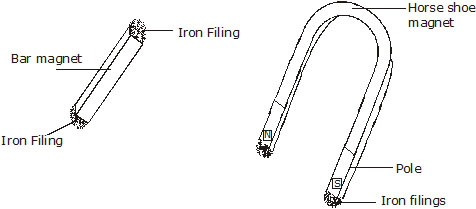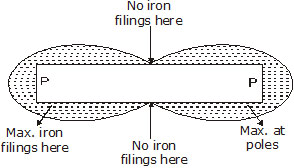Repeat the experiment with a horse-shoe magnet You will find that all magnets have maximum attractive power at their poles. This activity shows another fact about the attractive property of magnets.

(E) Directional Property: The end of the magnet that points towards the North is called the North Pole (N-Pole) and the other end of the magnet pointing towards the South is called the South Pole (S-Pole), A magnet always points in the north-south direction when suspended freely.

Activity
Take a bar magnet and suspend it freely as shown in figure or float it on a block of wood in water. The magnet comes to rest after sometime. Disturb it a little from its position of rest and see what happens. You will find that the magnet always comes to rest in the north-south direction.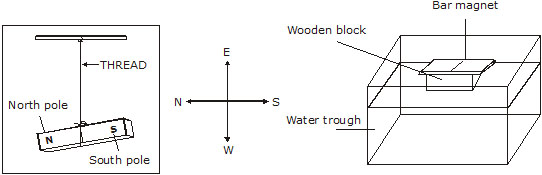(F)    Like poles repel each other:

Activity
Take two bar magnets. Suspend one magnet with a piece of thread. it will come to rest in the north-south direction. Hold the other magnet in your hand and bring its north pole near the north pole of the suspended magnet, as shown in figure. What do you see? You will find that the north pole of the suspended magnet is repelled, i.e., north pole moves away.
Now bring the south pole of the magnet in the hand near the north pole of the suspended magnet (see figure) You will find that the north pole of the suspended magnet will be attracted towards the south pole of the other magnet, i.e., north pole of the suspended magnet will come close to the south pole of the magnet in hand.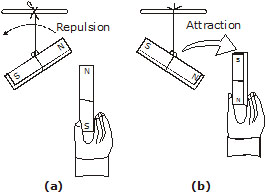We, therefore, conclude from this activity that like poles repel and unlike poles attract each other.

(G) Magnetic poles always exist in pairs: If a bar magnet broken into two pieces you will see that each piece behaves as a whole magnet. This shows that new poles are formed at the broken ends as shown in the figure. If these pieces are broken again, each smaller piece still remains a whole magnet with two opposite poles. Even a very small piece of a magnet is a whole magnet. Thus, we see that even the smallest piece of a magnet has north and south poles and we cannot separate the two poles.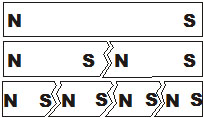We, therefore, conclude that the poles of a magnet cannot be separated. Magnetic poles always exist in pairs.

## MAGNETIC FIELD LINES

Magnetic field line is an imaginary line such that tangent to it at any paint gives the direction of magnetic field at that point in space. Magnetic field lines are drawn to represent magnetic field. Magnetic field lines can be drawn with the help of magnetic compass. Magnetic field lines are also called as magnetic lines of force.

### Properties of Magnetic field lines:

(i)    Magnetic field lines are close curves that start from north pole and end on south pole outside magnet. Inside magnet the field lines start from south pole and end on north pole.
(ii)    No two magnetic field lines ever intersect because if it is so, there will be two directions of magnetic field at that point which is not possible.
(iii)    Magnetic field lines come closer to one another near the pole of a magnet but they are widely separated at other places.

### Plotting magnetic field lines of a magnet:

To trace the magnetic field lines, place the bar magnet NS on a sheet of paper and mark its boundary. Mark a point A near the north pole of the given magnet. Place the compass needle (Figure) such that one of its ends (south) lies exactly over point A . Mark point B on the paper at the opposite (north) end of the compass needle. Move the compass needle so that the south end of the compass needle lies over B and mark point C at the north end of the needle and so on. Go on doing so till a point is reached near the south pole of the given magnet. Join all these points with a free hand curve so as to form a smooth dotted curve.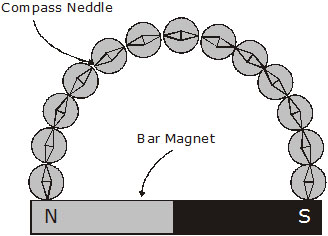Mark an arrow head to show the direction of magnetic field line, which will be north pole to south pole outside the magnet. This dotted curve marked with an arrow head represents a magnetic field line. Similarly starting from other points near the north pole of the magnet, draw other magnetic field lines. Magnetic field lines plotted for a bar magnet are as shown in figure.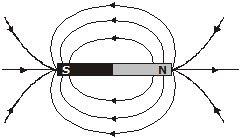We can visualise the magnetic field around a bar magnet by sprinking some iron filings near a bar magnet and tapping the sheet on which the magnet is placed. The iron filings will orient themselves according to figure.

### Magnetic Field of Earth

A freely suspended magnet always points in the north south direction even in the absence of any other magnet. This suggests that the earth itself behaves as a magnet which causes a freely suspended magnet or magnetic needle to point always in particular direction; north and south. The shape of the earth’s magnetic field resembles that an imaginary bar magnet.
The axis of earth magnetic field is inclined at an angle of about 15° with the geographical axis. Due to this a freely suspended magnet (or magnetic needle) makes an angle of about 15° with the geographical axis and points only approximately in the north south directions at a place.

### Magnetic Effect of Current (or Electromagnetism) :

The magnetic effect of current was discovered by Oersted in 1820. Oersted found that a wire carrying a current was able to deflect a compass needle. Now, the compass needle is a tiny magnet, which can be deflected only by a magnetic field. Since a current carrying wire was able to deflect a compass needle, it was concluded that a current flowing in a wire always gives rise to a magnetic field around it. The importances of magnetic field of current lies in the fact that gives rise to mechanical forces.

(i)    Magnetic field patterns produced by current carrying conductors having different shapes:
The pattern of magnetic field (or shape of magnetic field lines) produced by a current carrying conductor depends on its shape.
Different magnetic field patterns are produced by current carrying conductors having different shapes.
(A)    A straight conductor (or straight wire) carrying current.
(B)    A circular loop (or circular wire) carrying current.
(C)    A solenoid (long coil of wire) carrying current.

### (A)    Magnetic field pattern due to straight current carrying conductor (Straight current carrying wire):

The magnetic field lines around a straight conductor (straight line) carrying current are concentric circles whose centres lie on the wire. The magnetic field lines are circular in nature.
The magnitude of magnetic field produced by a straight current carrying wire at a given point is: (a) directly proportional to the current passing in the wire.
(b) inversely proportional to the distance of that point from the wire.

Direction of Magnetic Field Produced by current carrying conductor :

(a) According to Maxwell’s right hand thumb rule:
Imagine that you are grasping (or holding) the current carrying wire in your right hand so that your thumb points in the direction of current, then the direction in which your fingers encircle the wire will give the direction of magnetic field lines around the wire.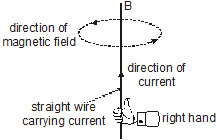Maxwell’s right hand thumb rule is also known as Maxwell’s corkscrew rule (Corkscrew is a device for pulling corks from bottles, and consists of a spiral metal rod and a handle).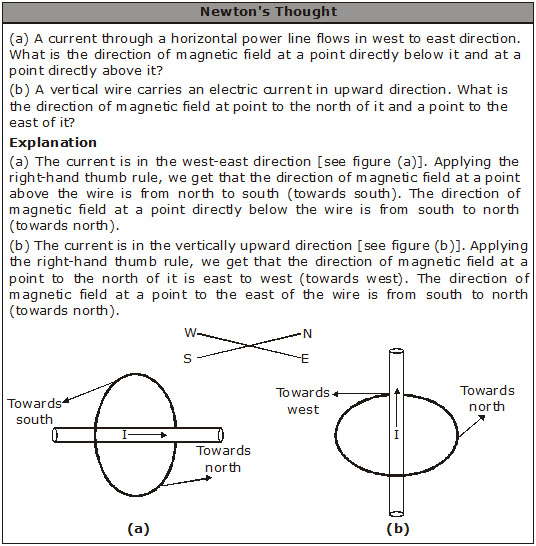(b) According to Maxwell’s corkscrew rule :
Imagine driving a corkscrew in the direction of current, then the direction in which we turn its handle is the direction of magnetic field (or magnetic field lines).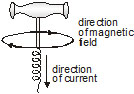### (B)     Magnetic field pattern due to a circular loop (or circular wire) Carrying current :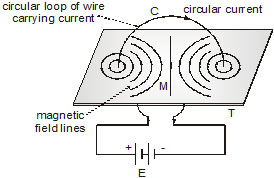When current is passed through a straight wire, a magnetic field is produced around it. It has been found that the magnetic effect of current increases if instead of using a straight wire, the wire is converted into a circular loop. When a current is passed through the circular loop of wire, a magnetic field is produced around it.

### The magnitude of magnetic field produced by a current carrying circular loop (or circular wire) at its centre is :

(i)    Directly proportional to the current passing through the circular loop (or circular wire), and
(ii)    Inversely proportional to the radius of circular loop (or circular wire).
The strength of magnetic field produced by a circular coil carrying current is directly proportional to both, number of turns (n), and current (I) ; but inversely proportional to its radius (r).
The strength of magnetic field produced by a current carrying circular coil can be increased -
(i) by increasing the number of turns of wire in the coil
(ii) by increasing the current flowing through the coil and,
(iii) by decreasing the radius of the coil.

### (C)     Magnetic field due to a solenoid :

The solenoid is a long coil containing a large number of close turns of insulated copper wire.
The magnetic field produced by a current carrying solenoid is similar to the magnetic field produced by a bar magnet.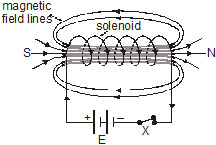The strength of magnetic field produced by a current carrying solenoid depends on :
(i)    The number of turns in the solenoid : Larger the number of turns in the solenoid greater will be the magnetism produced.
(ii)    The strength of current in the solenoid : Larger the current passed through solenoid, stronger will be the magnetic field produced.
(iii) The nature of core material used in making solenoid : The use of soft iron rod as core in a solenoid produces the strongest magnetism.

### Electromagnet

An electric current can be used for making temporary magnet known as electromagnets. An electromagnet works on the magnetic effect of current. An electromagnet consists of a long coil of insulated copper wire would on a soft iron core.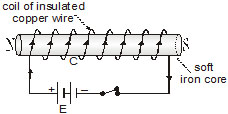When the two ends of the copper coil are connected to a battery, an electromagnet is formed. The core of an electromagnet must be of soft iron because soft iron loses all of its magnetism when current in the coil is switched off. If steel is used for making the core of an electromagnet, the steel does not lose all its magnetism when the current is stopped and it becomes a permanent magnet so steel isnot used for making electromagnets.

(a) Factors Affecting the Strength of an Electromagnet :
(i)    The number of turns in the coil : If we increase the number of turns in the coil, the strength of electromagnet increases.
(ii)    The current flowing in the coil :  If the current in the coil is increased, the strength of electromagnet increases.
(iii)The length of air gap between its poles : If we reduce the length of air gap between the poles of an electromagnet, then its strength increases.

### Magnetism in Human beings

Extremely weak electric current are produced in the human body by the movement of charged particles called ions. These are called ionic currents. When the weak ionic currents flow along the nerve cells, they produce magnetic field in our body. The two main organs of the human body where the magnetic field produced is quite significant are the heart and the brain.
The magnetism produced inside the human body (by the flow of ionic currents) forms the basis of a technique called Magnetic Resonance Imaging (MRI) which is used to obtain images (or pictures) of the internal parts of our body.

###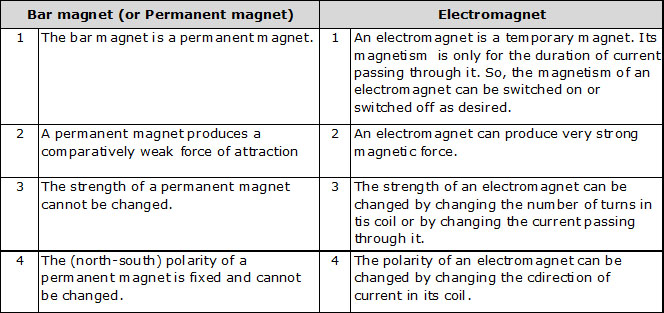Fleming’s Left hand Rule for the direction of Force

*   According to Fleming’s left hand rule :
Hold the forefinger the centre finger and the thumb of your left hand at right angles to one another. Adjust your hand in such a way that the forefinger points in the direction of magnetic field and the centre finger points in the direction of current, then the direction in which thumb points, gives the direction of force acting on the conductor.

###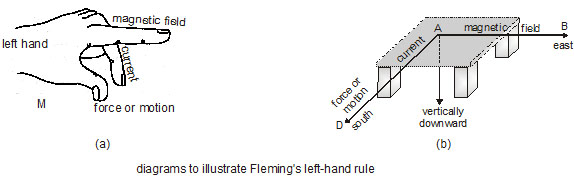Electric Motor

A motor is a device which converts electrical energy into mechanical energy. A common electric motor works on direct current, called D.C. motor, which means a ‘Direct Current Motor’.
(i)    Principle of a Motor : A motor works on the principle that when a rectangular coil is placed in a magnetic field and current is passed through it, a force acts on the coil which rotates it continuously.
(ii)    Construction of a Motor :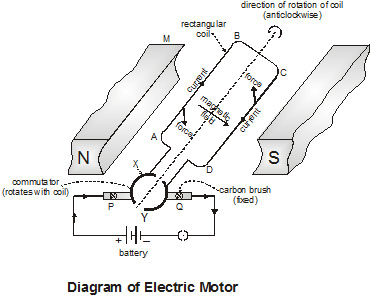An electric motor consists of a rectangular coil ABCD of insulated copper wire, which is mounted between the curved poles of a horseshoe - type permanent magnet M. The sides AB and CD of the coil are kept perpendicular to the direction of magnetic field between the poles of the magnet.
A device which reverses the direction of current through a circuit is called a commutator. The two ends of the coil are soldered permanently to the two half rings X and Y of a commutator. A commutator is a copper ring split into two parts X and Y, these two parts are insulated from one another and mounted on the shaft of the motor.
The commutator rings are mounted on the shaft of the coil and they also rotate when the coil rotates. The function of commutator rings is to reverse the direction of current flowing through the coil every time the coil just passes the vertical position during a revolution.
The carbon brushed P and Q are fixed to the base of the motor and they press lightly against the two half rings of the commutator. The battery to supply current to the coil is connected to the two carbon brushes P and Q through a switch.
The function of carbon brushes is to make contact with the rotating rings of the commutator and through them to supply current to the coil.

(iii)    Working of a Motor : When an electric current is passed into the rectangular coil, produces a magnetic field around the coil. Suppose that initially the coil ABCD is in the horizontal position.
The current flows in the direction ABCD and leaves via ring Y and  carbon brush Q.

(a)    In the side AB of the rectangular coil ABCD, the direction of current is from A to B. And in the side CD of the coil, the direction of current is from C to D.
By applying fleming’s left hand rule to sides AB and CD of the coil we find that the force on side AB of the coil is in the downward direction whereas the force on side CD of the coil is in the upward direction. Due to this the side AB of the coil is pushed down and side CD of the coil pushed up. The coil ABCD rotate in the anti clockwise direction.

(b)    While rotating, when the coil reaches vertical position, then the brushes P and Q will touch the gap between the two commutator rings and current to the coil is cut off. The coil does not stop rotating because it has already gained momentum due to which it goes beyond the vertical position.

(c)    After half rotation, when the coil goes beyond vertical position, the side CD of the coil comes on the left side whereas side AB of the coil comes to the right side, and the two commutator half rings automatically change contact from one brush to the other. So after half rotation of the coil, the commutator half ring Y makes contact with brush P whereas the commutator half ring X makes contact with brush Q. This reverses the direction of current in the coil. Due to this side CD of the coil is pushed down and the side AB of coil is pushed up. This makes the coil rotates anticlockwise by another half rotation.

(d)    The reversing of current in the coil is repeated after every half rotation due to which the coil continue to rotate as  long as current from the battery is passed through it. The rotating shaft of electric motor can drive a large number of machines which are connected to it.

### Electromagnetic Induction

(i)    Electricity From Magnetism :  The production of electricity from magnetism is called electromagnetic induction. The current produced by moving a straight wire in a magnetic field (or by moving a magnet in a coil) is called induced current.
The Phenomenon of electromagnetic induction was discovered by a British Scientist Michael Faraday and an American scientist Joseph Henry independently in 1831. The process of electromagnetic induction has led to the construction of generators for producing electricity at power stations. A galvanometer is an instrument which can detect the presence of electric current in a circuit.

(ii)     To demonstrate electromagnetic induction by using a coil and a bar magnet :
We have fixed coil of wire AB. The two ends of the coil are connected to a current detecting instrument called galvanometer.
When a bar magnet is held standstill inside the hollow coil of wire, there is no deflection in the galvanometer pointer showing that no electric current is produced in the coil.
When a bar magnet is moved quickly into a fixed coil of wire AB, then a current is produced in the coil. This current causes a deflection in the galvanometer pointer.
The production of electric current by moving a magnet inside a fixed coil of wire is also a case of electromagnetic induction. The condition necessary for the production of electric current by electromagnetic induction is that there must be a relative motion between the coil of wire and a magnet.

(a)    A current is induced in a coil when it is moved (or rotated) relative to a fixed magnet.
(b)    A current is also induced in a fixed coil when a magnet is moved (or rotated) relative to the fixed coil.
(c)    No current is induced in a coil when the coil and magnet both are stationary relative to one another.
(d)    When the direction of motion of coil (or magnet) is reversed, the direction of current induced in the coil also gets reversed.
(e)    The magnitude of current induced in the coil can be increased :
By winding the coil on a soft iron core,
By increasing the number of turns in the coil,
By increasing the strength of magnet, and
By increasing the speed of rotation of coil (or magnet).

### Fleming’s Right Hand Rule for the direction of induced current

(i)     According to fleming’s right hand rule : Hold the thumb, the forefinger and the centre finger of your right hand at right angles to one another. Adjust your hand in such a way that forefinger points in the direction of magnetic field and thumb point s in the direction of motion of conductor, then the direction in which centre finger points, gives the direction of induced current in the conductor.

### Electric Generator

The electric generator converts mechanical energy into electrical energy. A small generator is called a dynamo.
(i)  Principle of Electric Generator :  The electric generator works on the principle that when a straight conductor is moved in a magnetic field, then current is induced in the conductor.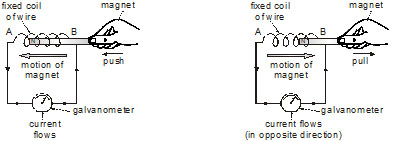(ii)  Electric generators are of two types :
(a) Alternating current generator (A.C. generator)
(b) Direct Current generator (or D.C. generator)

### (a) A.C. Generator :

Construction of an A.C. Generator :  A simple A.C. generator consists of a rectangular coil ABCD which can be rotated rapidly between the poles N and S of a strong horseshoe type permanent magnet M. The coil is made of a large number of turns of insulated copper wire.
The two ends A and D of the rectangular coil are connected to two circular pieces of copper metal called slip rings R1 and R2. As the slip rings R1 and R2 rotate with the coil, the two fixed pieces of carbon called carbon brushes, B1 and B2 keep contact with them.
The outer ends of carbon brushes are connected to a galvanometer to show the flow of current in the external circuit.

### Working of an A.C. generator :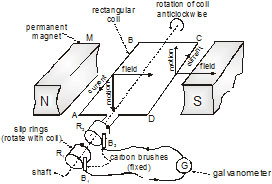Suppose that the generator coil ABCD is initially in the horizontal position.

(i)  As the coil rotates in the anticlockwise direction, the side AB of the coil moves down cutting the magnetic field lines near the N pole of the magnet, and side CD moves up, cutting the magnetic field lines near the S-pole of the magnet. Due to this, induced current is produced in the sides AB and CD of the coil. On applying fleming’s right hand rule to the sides. AB and CD of the coil, we find that the currents are in the directions B to A and D to C. Thus, the induced currents in the two sides of the coil are in the same direction. Thus, in the first half revolution of coil, the current in the external circuit flows from brush B1 to B2.

(ii)  After half revolution, the sides AB and CD of the coil will interchange their positions. The side AB will come on the right hand side and side CD will come on the left side.
So, after half a revolution, side AB starts moving up and side CD starts moving down.

As a result of this, the direction of induced current in each side of the coil is reversed after half a revolution giving rise to the net induced current in the direction CDAB. The current in the external circuit now flows from brush B2 to B1. Thus, in 1 revolution of the coil, the current reverse its direction 2 times. In this way alternating current is produced in this generator.

(iii) The alternating current (A.C.) produced in India has a frequency of 50 Hz. A.C. generators are used in power stations to generates electricity.

### (b) D.C. Generator :

In order to obtain direction current (which flows in one direction only), a D.C. generator is used Actually, If we replace the slip rings of an A.C. generator by a commutator, then it will become a D.C. generator. Thus, in a D.C. generator, a split ring type commutator is used (like the one used in an electric motor). When the two half rings of commutator are connected to the two ends of the generator coil, then one carbon brush is at all times in contact with the coil arm moving down in the magnetic field while the other carbon brush always remains in contact with the coil arm moving up in the magnetic field. Due to this, the current in outer circuit always flows in one direction. So, it is direct current.

###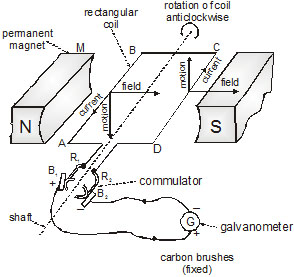Domestic Electric Circuit (or Domestic Wiring)

Electric power is usually generated at places which are very far from the places where it is consumed. At the generating station, the electric power is generated at 11,000 volt (because voltage higher than this causes) insulation difficulties, while the voltage lower than this involves high current). This voltage is alternating of frequency 50 Hz. (i.e. changing its polarity 50 times in a second). The power is transmitted over long distances at high voltage to minimise the loss of energy in the transmission line wires. For a given electric power, the current becomes low at a high voltage and therefore the loss of energy due to heating (= I2 Rt) becomes less. thus, the alternating voltage is stepped up from 11 kV to 132 kV at the generating station (called grid sub station). It is then transmitted to the main sub station. At the main sub station, this voltage is stepped down to 33 kV and is transmitted to the switching transformer station or the city sub station. At the city sub station, it is further stepped down to 220 kV for supply to the consumer as shown in figure.
To supply power to a house either the overhead wires on poles are used or an underground cable is used before the electric line is connected to the meter in a house, a fuse of high rating (» 50 A) is connected a the pole or before the meter. This is called the company fuse. The cable used for connection has three wires (i) live (or phase) wire, (ii) neutral wire, (iii) earth wire. The neutral and the earth wires are connected together at the local sub station, so the neutral wire is at the earth potential. After the company fuse, the cable is connected to a kWh meter. From the meter, connections are made to the distributions board through a main fuse and a main switch.
The main switch is a double pole switch. It has iron covering. The covering is earthed. This switch is used cut the connections of the live as well as the neutral wires simultaneously. The main switch and the meter are locally earthed (in the compound of house). From the distribution board, the wires go to the different part of the house.

### Electric Fuse

The electric wires used in domestic wiring are made of copper metal because copper is a good conductor of electricity having very low resistance.
If the current passing through wires exceeds this maximum value, the copper wires gets over heated any may even cause a fire. An extremely large current can flow in domestic wiring under two circumstances: short circuiting and overloading.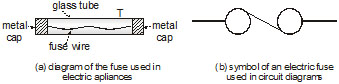(i)    Short circuiting : If the plastic insulation of the live wire and neutral wire gets torn, then the two wires touch each other. This touching of the live wire and neutral wire directly is known as short circuit.

(ii)    Overloading : If too many electrical appliances of high power rating are switched on at the same time, they draw an extremely large current from the circuit. This is known as overloading the circuit. Overloading can also occur if too many appliances are connected to a single socket.
A fuse is a safety device having a -short length of a thin, tin plated copper wire having low melting point, which melts and breaks the circuit if the current exceeds a safe value.
An electric fuse works on the heating effect of current. A fuse wire is connected in series in the electric circuit.

### SOLVED PROBLEMS :-

Ex.1 Give some basic properties of magnets :
Sol.    Some basic properties of magnets are as follows :
(i)     Attractive property :
A magnet attracts small pieces of iron, cobalt, nickel, etc.
(ii)     Directive property :
A freely suspended magnet aligns itself nearly in the north-south direction.
(iii)     Law of magnetic poles :
Like magnetic poles repel and unlike magnetic poles attract each other.
(iv)    Magnetic poles exist in pairs :
If we break a magnet  into two pieces, we always get two small dipole magnets. It is not possible to obtain an isolated N-pole or S-pole.

Ex.2  What are magnetic lines of force. Give their important properties :
Sol.  Magnetic lines of force :  A magnetic line of force may be defined as the curve the tangent to which at any point gives the direction of the magnetic  field at that point. It may also be defined as the path along with a free north pole                                           tends to move.
Properties of  lines of force :
(i)      These are closed curves which start in air from the N-pole and end at the S-pole or i then return to the N-pole through the interior of the magnet.
(ii)    No two magnetic  lines of force can intersect each other.
(iii)    They start from and end on the surface of the magnet normally.
(iv)    The lines of force have a tendency to contract lengthwise and expand sidewise. This explains attraction between unlike poles and repulsion between like poles.
(v)    The relative closeness of the lines of force gives a measure of the strength of the magnetic field which is maximum at the poles.

Ex.3    Name and state the two rules for finding the direction of magnetic field produced by electric current through a straight conductor.
Sol.    The direction of the magnetic field produced by a current carrying straight conductor can be obtained by using any of the following two rules :
(i)    Right hand thumb rule :If the current carrying conductor is held in the right hand such that the thumb points in the direction of the current, then the direction of the curl of the fingers will give the direction of the magnetic field, as shown in figure.
(ii) According to Maxwell’s corkscrew rule :Imagine driving a corkscrew in the direction of current, then the direction in which we turn its handle is the direction of magnetic field (or magnetic field lines).

Ex.4    A straight conductor is held perpendicular to the plane of paper and it carries a current (a) upwards, (b) downwards, Draw the magnetic lines of force ?
Sol.    (a) See figure (a) Lines of force have anticlockwise sense.
(b) See figure (b) Lines of force clockwise sense.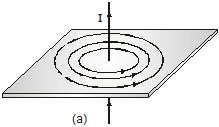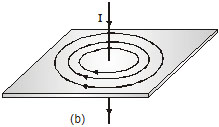Ex.5    Draw and discuss the pattern of the magnetic lines of force of a current carrying circular loop.
Sol.    Magnetic field due to a current through a circular loop :
The magnetic field lines of  a circular wire carrying a current. The lines of force near the wire are almost concentric circles. As we move towards the centre of loop, the concentric circles become larger and larger. Near the centre  of the loop, the areas of these big circles appear as parallel straight lines.
Thus the magnetic field is almost uniform at the centre of the loop. By applying right hand rule, we can see that the magnetic  field lines due to all sections of the wire are in the same direction within the loop.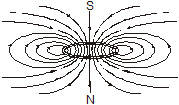Ex.6    (a)     What is a solenoid ?
(b)     Draw a rough sketch of the pattern of the filed lines due to a solenoid carrying current?
(c)     Compare the magnetic behaviour of a straight solenoid with that of a bar magnet ?
(d)     ON what factors does the strength of the magnetic field produced by a current carrying solenoid depend?
Sol.    (a)     Solenoid : A long cylindrical coil of insulated copper wire of large number of circular turns is  called a solenoid.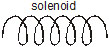(b)     When an electric current is passed through a solenoid, it produces a magnetic field around it Its magnetic field pattern is shown in figure.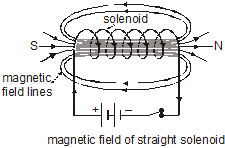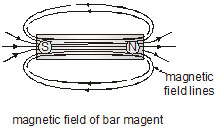(c)    When a current is passed through the solenoid, the current in each circular loop has the same direction, their magnetic effects get added up producing a strong magnetic field.
Inside the solenoid, the magnetic field is almost uniform and parallel to the axis of the solenoid.
The magnetic field produced by a solenoid  is very much, similar to that of magnet like a bar magnet, one end of the solenoid has N polarity while her end has S-polarity.
The polarity of any end (face)  of the coil can be determined by clock rule.
For all practical purposes, the magnetic field of a solenoid and that of a bar at can be taken  identical.
(d)     Factors on which the strength of the magnetic field produced by a current solenoid
depends :
(i)     Number of turns in the solenoid :
The larger the number of turns in the stronger is the magnetic field produced.
(ii)     Strength of the current :
The larger the current passed through the solenoid is the magnetic field produced.
(iii)     Nature of the core material :
By winding the coil over a soft iron cylinder, core, the magnetic field can be increased several        thousands times.

Ex.7    What is an electromagnet ? On factors does the strength of an electromagnet depend ?
Sol.    Electromagnet :
A soft iron core placed inside a solenoid behaves like a powerful when a current is passed through a solenoid. This device is called an electromagnet.
The current is switched of, the iron core loses its magnetism and so it is no longer an electromagnet. Thus, electromagnet are temporary magnets.
Factors on which the strength of an electromagnet depends :
(i)     Number of turns in the coil  :
The larger the number of turns in the coil, is the strength of the electromagnet.
(ii)    Strength of the current :
The larger the current passed through the solenoid, powerful is the electromagnet.
(iii)    Nature of the core material :
The core of the magnetic material like soft iron the strength of the electromagnet.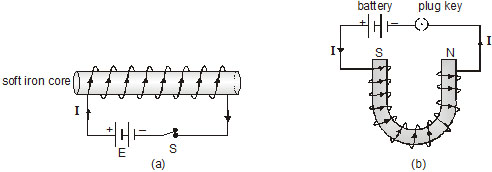Ex.8    Name and state the rule to determine the direction of a force experienced by a straight conductor carrying current placed in a magnetic field which is perpendicular to it.
Sol.    Fleming’s left hand rule. Stretch the forefinger, the central finger and the thumb of the left hand mutually perpendicular to each other.
If the forefinger points in the direction of the magnetic field, the central finger in the direction of current, then the thumb points in the direction of force on the conductor.

Ex.9    Name and define the SI unit of magnetic field.
Sol.    SI unit of magnetic field is tesla : (T)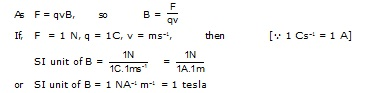So, one tesla is that magnetic field in which a charge of one coulomb moving with a velocity of 1 m/s perpendicular to the magnetic field experience a force  one newton.

Ex.10    With the help of a labelled diagram, explain the principle, construction and working of an electric motor. What is the function of a split ring in an electric motor ?
Sol.    Electric motor : We describe here a d.c. motor which operates on direct current obtained from a battery.
Principle  : An electric motor works on the principle that a current carrying conductor placed in a magnetic field experiences a force, the direction of force is given by Fleming’s left hand rule.
After half a rotation, as shown in Figure the split ring S1 comes in contact with bush B2 and S2 in contact with brush B1.
Therefore, the current in the coil gets reversed and flows along the path DCBA.
A device that reverse the direction of flow of current through a circuit is called commutator. In electric motors, the split ring acts as a commutator.
Thus the arm AB is now pushed up and the arm CD is pushed down.
Therefore, the coil and the axis rotate half a turn more in the same direction.
The reversing of the current is repeated at each half rotation, giving rise to a continuous rotation of the coil and to the axis.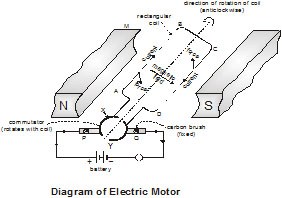### * NCERT QUESTIONS WITH SOLUTIONS :-

Q.1    Why does a compass needle get deflected when brought near a bar magnet ?
Ans.    Compass needle is a small magnet which experiences a force in the magnetic field of a bar magnet. Due to this force, it gets deflected.

Q.2    Draw magnetic field lines around a bar magnet.
Ans.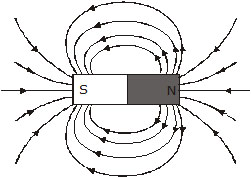Q.3    List the properties of magnetic lines of force.
Ans.    (i)  Magnetic lines of force are closed continuous curves.
(ii)     The tangent at any point on the magnetic line of force gives the direction of the magnetic field at that point.
(iii)     Two magnetic lines of force never cross each other.

Q.4    Why do no two magnetic lines of force intersect each other?
Ans.    The tangent at any point on a magnetic field line gives the direction of magnetic field at that point. If two magnetic field lines cross each other, then at the point of intersection, there will be two tangents. Hence, there will be two directions of the magnetic field at the point of intersection. This is not possible. Hence, no two magnetic field lines can cross each other.

Q.5    Consider a circular loop of wire lying in the plane of the table. Let the current pass through the loop clockwise. Apply the right hand rule to find out the direction of magnetic field inside and outside the loop.
Ans.    Magnetic field inside the loop is perpendicular to the plane of table and in the downward direction. Outside the loop, magnetic field is perpendicular to the plane of the table and in the upward direction.

Q.6    The magnetic field in a given region is uniform. Draw a diagram to represent it.
Ans.
Q.7    Choose the correct option. The magnetic field inside a long straight solenoid-carrying current
(A) is zero
(B) decreases as we move towards its ends
(C) Increases· as we move towards its ends.
(D) is the same at an points.
Ans.    The magnetic field Inside a long straight solenoid-carrying current decreases as we move towards its ends. At the ends of solenoid, the strength of the magnetic field is almost the half that In the middle of the solenoid.Thus, the correct option is (B).

Q.8    Which of the property a proton can change when it moves freely in a magnetic field? (There may be more than one correct answer)
(A) mass         (B) speed        (C) velocity         (D) momentum.
Ans.    Motion of a charged particle like proton in a magnetic field is a circular path. Hence its velocity and momentum can change. Thus, option (B) and (D) are correct.

Q.9    In activity physics 12.7 (in NCERT), how do we think the displacement of rod PQ will be affected if (i) current in rod PQ is increased (ii) a stronger horseshoe magnet is inserted (iii) length of the rod PQ is increased.
Ans.    (i) When current in rod increases, force on the rod also increases. Hence, the displacement of the rod increases.
(ii) When a stronger horseshoe magnet is inserted, magnetic field increases. Thus, force on the rod also increases. Hence, displacement of the rod increases.
(iii) When length of the rod increases, force on the rod also increases and hence, displacement increases.

Q.10    A positively charged particle (alpha particle) projected towards west is deflected towards north by a magnetic field. The direction of magnetic field is
(A) towards south        (B) towards east        (C) downward            (D) upward
Ans.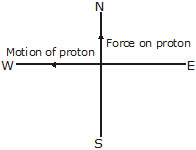By applying Fleming’s left hand rule, we find that the magnetic field is in upward direction. Thus, option (D) is correct.

Q.11    State fleming's left hand rule.
Ans.    'Stretch the thumb, forefinger and central finger of your left hand such that they are mutually perpendicular. If the fore finger points in the direction of magnetic field and the central finger in the direction of current, then the thumb will point in the direction of motion or the force acting on the conductor'.

Q.12    What is the principle of electric motor?
Ans.     It is based on the principle that a current carrying coil experiences equal and opposite forces, on its edges which rotates it continuously.

Q.13    What is the role of the split ring in an electric motor?
Ans.    The split-ring in an electric motor reverses the direction of current in the coil of the motor. Therefore, the direction of the force acting on the two arms of the coil is also reversed. As a result of this, the coil of d.c. motor continues to rotate in the same direction.

Q.14    Explain different ways to induce current in a coil.
Ans.    (i) By moving a bar magnet towards or away from the coil.
(ii) By placing a coil near another coil which carries a variable current.

Q.15    State the principle of electric generator.
Ans.    It is based on the principle of electromagnetic induction. That is, the changing magnetic field induces current in the coil.

Q.16    Name some sources of direct current.
Ans.    A dry cell, a battery, a solar cell, d.c. generator, etc. are some sources of direct current.

Q.17    Which source produces alternating current?
Ans.    AC generator is the source which produces alternating current.

Q.18    Choose the correct option. A rectangular coil of copper wires is rotated in a magnetic field. the direction of the induced current changes once in each
(A) two revolutions                    (B) one revolution
(C) half revolution                    (D) one-fourth revolution
Ans.    The direction of induced current changes twice in one revolution i.e., it changes after every half revolution. Thus, option (C) is correct.

Q.19    Name two safety measures commonly used in electric circuit and appliances.
Ans.    (i) Electric fuse        (ii) Earthing (or grounding).

Q.20    An electric oven of 2kW power rating is operated in a domestic electric circuit (220V) that has a current rating of 5A. What result do you expect? Explain.
Ans.    Power, P = 2kW = 2000W; V = 220V
No, P = V × I        or    I = P/V
or I = (2000)/220 = 9.09 A.
This shows that current flowing through, the oven is more than the current rating (5A). Hence, the fuse in the circuit melts i.e., the circuit breaks preventing the oven from damage.

Q.21    What precautions should be taken to avoid the overloading of domestic electric circuit ?
Ans.    (i) We should not connect many appliances in the same socket.
(ii) Electrical appliances of high power rating should not be switched on simultaneously.
(iii) Electric wires with good quality insulating covers should be used to prevent the short Circuiting.

Q.22    Which of the following correctly describes the magnetic field near a long straight wire ?
(A) the field consists of straight lines perpendicular to the wire.
(B) the field consists of straight lines parallel to the wire.
(C) the field consists of radial lines originating from the wire.
(D) the field consists of concentric circles centered on the wire.
Ans.    The magnetic field lines around a straight current carrying conductor are concentric circles with the conductor located at their centre. Thus, the option (D) is correct.

Q.23    The phenomenon of electromagnetic induction is
(A) the process of charging a body
(B) the process of generating magnetic field due to current passing through a coil.
(C) producing induced current in a coil due to relative motion between a magnet and the coil.
(D) the process of rotating a coil of an electric motor.
Ans.    Here, option (C) is correct.

Q.24    The device used for producing electric current is called a
(A) generator         (B) galvanometer        (C) ammeter         (D) motor
Ans.    Option (A) is correct.

Q.25    The essential difference between an AC generator and a DC generator is that
(A) AC generator has an electromagnet while a DC generator has permanent magnet.
(B) DC generator will generate a higher voltage
(C) AC generator will generate a higher voltage
(D) AC generator has slip rings while the DC generator has a commutator.
Ans.    An AC generator has slip rings while a DC generator has split ring commutator. Thus, option (D) is correct.

Q.26    At the time of short circuit, the current in the circuit
(A) reduces substantially                (B) does not change
(C) increases heavily                    (D) varies continuously
Ans.    Short circuit takes place when the live wire and the neutral wire come into direct contact, the resistance in the circuit becomes very low and the current in the circuit abruptly increases. Thus, option (C) is correct.

Q.27    State whether the following statements are true or false.
(A) An electric motor converts mechanical energy into electrical energy.
(B) An electric generator works on the principle of electromagnetic induction.
(C) The field at the centre of a long circular coil carrying current will be parallel straight lines.
(D) A wire with a green insulation is usually the live wire.
Ans.    (A) False. It converts electrical energy into mechanical energy.
(B) True.
(C) True.
(D) False. Live wire has red insulation cover.

Q.28    List three sources of magnetic fields.
Ans.    (i) a permanent magnet.        (ii) a current carrying solenoid.
(iii) Current carrying wire.

Q.29    How does a solenoid behave like a magnet? Can you determine the north and south poles of a current carrying solenoid with the help of a bar magnet. Explain.
Ans.    When electric current flows through a solenoid, magnetic field is set up around the solenoid. One end of the solenoid behaves as north pole and the other end of the solenoid behaves as south pole. To determine the north and south poles of a current carrying solenoid bring the north pole of a bar magnet towards one end of the solenoid. If the solenoid attracts towards the magnet, then that face of the solenoid is south pole. If the solenoid moves away from the bar magnet, then that face of the solenoid is the north pole.

Q.30    State the rule to determine the direction of a (i) magnetic field produced around a straight conductor carrying bar current, (ii) force experienced by a current-carrying straight conductor placed in a magnetic field which is perpendicular to it, and (iii) current induced in a coil due to its rotation in a magnetic field.
Ans.    (i) right hand thumb rule.
(ii) Fleming’s left hand rule.
(iii) Fleming’s right hand rule.

Q.31    When is the force experienced by a current carrying conductor placed in magnetic field the largest ?
Ans.    When current carrying conductor is placed perpendicular to the magnetic field.

Q.32    Think you are sitting in a chamber with your back to one wall. An electron beam moving horizontally from back wall towards the front wall, is deflected by a strong magnetic field to your right side. What is the direction of magnetic field?
Ans.    Movement from electron beam from back wall to the front wall is equivalent to the flow of electric current from front wall to the back wall. The deflection of the beam means, the force is acting towards our right side. According to Fleming’s Left Hand Rule, the direction of magnetic field is vertically downward.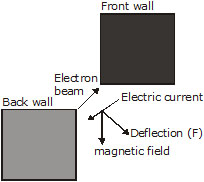Q.33    A coil of insulated copper wire is connected to a galvanometer. What will happen if a bar magnet is
(i) pushed into the coil (ii) withdrawn from inside the coil, (iii) held stationary inside the coil?

Ans.    (i) When a bar magnet is pushed into the coil, induced current flows through the coil due to the phenomenon of electromagnetic induction. This induced current is indicated by the deflection of the needle of the galvanometer inserted into the coil.
(ii) When a bar magnet is withdrawn from inside the coil, again induced current flows through the coil due to the phenomenon of electromagnetic induction. In this case, the direction of induced current is opposite to the direction of the current in case (i).
(iii) When the bar magnet is held stationary inside the coil, there is no change in magnetic field around the coil. Hence, no induced current flows through the coil. therefore, galvanometer shown no
deflection.

Q.34    Name some devices in which electric motors are used.
Ans.    Pump sets, electric cars, rolling mills, electric fans, hair drier, etc.

Q.35    Two circular coils A and B are placed close to each other. If the current in the coil A is changed, will some current be induced in the coil B? Given reason.
Ans.    When current in coil A is changed, a changing magnetic field is set up around it. this changing magnetic field also links with coil B and hence some current will be induced in coil B due to electromagnetic induction.

Q.36    When does an electric short circuit occur?
Ans.    when live wire and neutral wire touch each other (i.e. come in direct contact).

Q.37    What is the function of an earth wire? Why is it necessary to earth metallic casings of electric appliances.
Ans.    Earth wire acts as a safety measure. When the live wire touches the metallic casing of an electric appliance, the electric current flows from the casing of the appliance to the earth through the copper wire. An electric current flows in the direction of low resistance thus, current passes through the copper wire instead of human body. Thus, the human body is saved from electric shock.

Q.1    What is a magnet ?

Q.2    What is a natural magnet ?

Q.3    What is the meaning of the word lodestone?

Q.4    What is the origin of the word magnetism ?

Q.5    State the law of magnetic poles ?

Q.6    What is the cause of magnetism ?

Q.7    What happens if a bar magnet is cut into two pieces (i) transverse to its length (ii) along its length ?

Q.8    Are the two poles of a magnet equally strong?

Q.9    Define the term magnetic field ?

Q.10    Define a magnetic line of force ?

Q.11    Give two properties of magnetic lines of force

Q.12    Can two magnetic lines of force intersect ? Justify your answer.

Q.13    Magnetic lines of force are endless ? Comment

Q.14    A freely suspended magnet always points along north-south direction. Give reason ?

Q.15    Name the scientist who first established a connection between electricity and magnetism.

Q.16    What is magnetic effect of current ?

Q.17    State Oersted observation ?
Or
How can it be shown that a magnetic field exists around a wire throught which a direct electric current is passing ?

Q.18    What is the nature of the magnetic field associated with a straight current carrying conductor ?

Q.19    State the right hand rule for finding the direction of magnetic field of a straight current carrying conductor ?

Q.20    How can we locate a current carrying wire concealed in a wall ?

Q.21    What does an electric current carrying  wire behaves like?

Q.22    Define magnetic field?

Q.23    Define field lines?

Q.24    What kind of quantity is magnetic field?

Q.25    What is a compass needle?

Q.26    State Fleming’s left hand rule.

Q.27    State and explain how right hand thumb rule indicates magnetic field.

Q.28    Why do we use power supply of two different current ratings at our homes?

Q.29    What is an electric fuse? What material is selected for fuse wire?

Q.30    Give four features of domestic electric wiring.

Q.31    (a)    What is meant by a magnetic field?
(b)    How is the direction of magnetic field at a point determined?
(c)    What is the direction of magnetic field at  the centre of a current- carrying circular  loop.

Q.32    (a) What is an electromagnet? What does it consist of?
(b)    Name one material in each case which is used to make a
(i)    Permanent magnet
(ii)    Temporary magnet

Q.1    The fact that magnetic field is produced around a wire carrying a current, was discovered by
(A) Faraday     (B) Oersted     (C) Maxwell     (D) Joule.

Q.2    When the current is passing through the straight wire then, the associated magnetic field is
(A) Straight     (B) Elliptical     (C) Circular     (D) Parabolic.

Q.3    When current is circular, the associated magnetic field is
(A) Straight     (B) Elliptical     (C) Circular     (D) Parabolic.

Q.4    When current flows clockwise in a loop, the polarity of its face is
(A) East     (B) South     (C) West     (D) North.

Q.5    When current flows anticlockwise in a loop the magnetic polarity of the face is
(A) East     (B) South     (C) West     (D) North.

Q.6    For a solenoid carrying a current I and having n turns per unit length, wrapped on a core of permeability m, the correct expression for magnetic field intensity (B) is
(A)  B= nI    (B) B =     (C) B = m0mnI     (D) B =

Q.7    Magnets having temporary magnetism are called
(A) Electromagnets
(B) Bar magnets
(C) Circular magnets
(D) Horse-shoe magnets.

Q.8    Direction of force acting on a current carrying conductor kept in a magnetic field is given by
(A) Fleming's right hand rule     (B) Fleming's left hand rule
(C) Lenz's rule         (D) Faraday's rule.

Q.9    The electric device which works on the phenomenon of force on a current carrying conductor in a magnetic field is
(A) Generator     (B) Accelerator     (C) Motor     (D) Transformer.

Q.10    Electromagnetic induction was discovered by
(A) Oersted     (B) Maxwell.     (C) Thomson     (D) Faraday.

Q.11    Direction of induced current produced by motion of a conductor in a magnetic field is given by
(A) Fleming's right hand rule     (B) Fleming's left hand rule
(C) Lenz's rule         (D) Faraday's rule.

Q.12    In domestic electric circuits, fuse must be placed in series with
(A) Earth wire         (B) Neutral wire
(C) Live wire         (D) Any of the three wires.

Q.13    In a three pin socket (shoe) the bigger hole is connected to
(A) Any wire     (B) Live wire     (C) Neutral wire     (D) Earth wire.

(A) Less     (B) More     (C) Zero     (D) Not definite.

Q.15    Melting point of material of a fuse wire must be
(A) Moderate     (B) High     (C) Low     (D) Infinite.

Q.16    The phenomenon of electromagnetic induction is
(A) The process of charging a body
(B) The process of generating magnetic field due to a current passing through a coil
(C) Producing induced current in a coil by relative motion between a magnet and the coil
(D) The process of rotating a coil of an electric motor.

Q.17    The device used for producing electric current is called a
(A) Generator     (B) Galvanometer    (C) Ammeter     (D) Motor.

Q.18    At the time of short-circuit, the current in the circuit
(A) Reduces substantially         (B) Does not change
(C) Increases heavily         (D) Vary continuously.

Q.19     Two coil A and B placed close to each other. If current in the coil A is changed, some current will be induced in the coil B. This is because of electromagnetic induction. In this statement :
(A) Inference is correct but reasoning is not correct
(B)  Inference is incorrect but reasoning is correct
(C) Inference as well as reasoning are correct
(D)  Neither inference nor reasoning is correct.

Q.20    Which of the following property of a proton can change while it moves freely in a magnetic field?
(A) Mass     (B) Speed     (C) Velocity     (D) None of these

Q.21    The magnetic field inside a long straight solenoid carrying current
(A) Is zero         (B) Decreases as we move towards its end
(C) Increases as we move towards its end     (D) Is the same at all points.

Q.22    A magnetic field exerts no force on :
(A) An electric charge moving perpendicular to its direction
(B) an unmagnetised iron bar
(C) a stationary electric charge
(D) a magnet

Q.23    Which of the following instruments works by electromagnetic induction ?
(A) telephone receiver         (B) simple motor
(C) dynamo         (D) moving coil galvanometer.

Q.24    The use of fuses in electric power-lines is :
(A) to keep main voltage constant
(B) to open the line permanently when there is an overload
(C) just to show an indication when there is an overload
(D) as a switch to use in an emergency

Q.25    When the main switch of the house circuit is put off, it disconnects the :
(A) live wire         (B) neutral wire
(C) earth wire
(D) live and neutral wires.

Q.26    When a fuse is rated at 8 A, it means :
(A) it will not work if current is less than 8 A     (B) it has a resistance of 8 ohm
(C) it will work only if current is 8 A     (D) it will burn if current exceeds 8 A.

Q.27    According to International Convention of colour coding of wires used in household electrical circuits :
(A) live is red, neutral is black and earth is green
(B) live is red, neutral is green and earth is black
(C) live is brown, neutral is blue and earth is black
(D) live is brown, neutral is light blue and earth is green.

Q.28    Potential difference between a live wire and the neutral wire is :
(A) 150 V     (B) 210 V     (C) 200 V     (D) 220 V.

Q.29     Which of the following statements is incorrect regarding magnetic field lines :
(A) The direction of magnetic field at a point is taken to be the direction in which the north pole of a magnetic compass needle points
(B) Magnetic field lines are closed curves
(C) If magnetic field lines are parallel and equidistant, they represent zero field strength
(D) Relative strength of magnetic field is shown by the degree of closeness of the field lines

Q.30     Magnetic field lines determine :
(A) The shape of the magnetic field
(B) Only the direction of the magnetic field
(C) Only the relative strength of the magnetic field
(D) Both the direction and the relative strength of the magnetic field

Q.31     The magnetic field near a long straight wire is described by :
(A) Straight field lines parallel to the wire    (B) Straight field lines perpendicular to the wire
(C) Concentric circles centered on the wire    (D) Radial field lines starting from the wire

Q.32     Magnetic field inside a long solenoid carrying current is :
(A) Same at all points
(B) Minimum in the middle
(C) More at the ends than at the centre
(D) Found to increase from one end to the other.

Q.33    The frequency of direct current is :
(A) Zero    (B) 50 Hz    (C) 60 Hz    (D) 100 Hz.

Q.34     The frequency of household supply of a.c. in India is :
(A) Zero    (B) 50 Hz    (C) 60 Hz    (D) 100 Hz.

Q.35     The most important safety device method used for protecting electrical appliances from short circuiting or overloading is :
(A) Earthing
(B) Use of stabilizers
(C) Use of electric meter    (D) Use of fuse

Q.36     Current flowing in conductor A is 2A and current flowing in conductor B is 4A. The ratio of magnetic field produced around conductor A to the magnetic field produced around conductor B at a distance 10 cm from both the conductors is :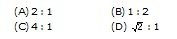Q.37     Current flowing in conductors A and B is same, what is the ratio of the magnetic field produced around the conductor A at a distance of 5 cm from the conductor to the magnetic field produced around the conductor B at a distance of 2 cm from this conductor is
(A) 0.04    (B) 0.4    (C) 4.0    (D) 10

Q.38     Same amount of current flows in the same direction along  the two parallel conductors separated by a small distance :
(A) Both conductors attract each other
(B) Both conductors repel each other
(C) Conductors neither attract each other nor repel each other
(D) Both conductors rotates about their axis.

Q.39     Magnetic field produced at the centre of a current carrying circular wire is :
(A) Directly proportional to the square of the radius of the circular wire
(B) Directly proportional to the radius of the circular wire
(C) Inversely proportional to the square of the radius of the circular wire
(D) Inversely proportional to the radius of the circular wire.

Q.40     Magnitude of magnetic field intensity at a point around a current carrying conductor is B. If the strength of current in the conductor becomes double, then the magnitude of magnetic field intensity at the point around the conductor is :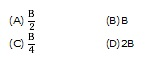Q.41     Two parallel conductor carrying current in the opposite directions
(A)  Repel each other
(B) Attract each other
(C) Sometimes attract and sometimes repel each            other
(D) None of these

Q.42    Force acting on a stationary charge Q in the magnetic field B is :
(A) B Q u    (B) BQ/u    (C) Bu/Q    (D) Zero

Q.43     A current carrying conductor placed perpendicular to the magnetic field experience a force. The displacement of this conductor in the magnetic field can be increased by.
(A) Decreasing the magnetic field    (B) Decreasing the current in the conductor
(C) Increasing the magnetic field    (D) Decreasing the length of the conductor.

Q.44     A conductor of length 50 cm, carrying current of 0.1 A, when placed perpendicular to direction of magnetic field 0.2 T experience force :
(A) 1.0 N
(B) 0.1 N
(C)0.01N
(D) 0.01 N.

Q.45     A charged particle having charge 1.6 × 10–19 C travels with a speed of 3.2 × 106 ms–1 in a direction parallel to the direction of magnetic field 0.04 T. The force experienced by the particle is :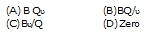Q.46     A magnetic compass is placed near a current carrying wire. The deflection of the needle of the magnetic compass increases. It shows that:
(A) Current in the wire is decreasing
(B) Current in the wire is increasing
(C) Current in the wire has nothing to do with the deflection of the needle of the magnetic compass
(D)  Magnetic compass has been disturbed by some one.

Q.47    A soft iron rod can be made an electro-magnet. Which of the following procedures would be adopted to test that the soft iron rod had become a magnet ?
(A) Place the iron rod near a bar magnet and observe if it attracts iron pins
(B) Place the iron rod near a current carrying wire and observe if it attracts iron pins
(C) Place the iron rod inside a current carrying solenoid and observe if it attracts iron pins
(D)  None of these.

Q.48    Current can be made to flow through a coil without connecting the coil with a battery. Which of the following procedures would be most suited to test this fact ?
(A) Connect a galvanometer across the ends of the coil and observe if galvanometer shows a deflection, when a bar magnet is placed near the one end of the coil.
(B) Connect a galvanometer across the ends of the coil and observe if galvanometer shows a deflection, when a bar magnet is placed inside the coil.
(C) Connect the galvanometer across the ends of the coil and observe if galvanometer shows a deflection, when a bar magnet is moved towards or away from the coil and along the axis of the coil.
(D) None of these

Q.49    An electron enters a magnetic field at right angles to it as shown in figure. The direction of force acting on the electron will be [NTSE]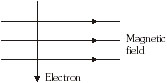(A) To the right
(B) To the left
(C) Out of the page
(D) Into the page.

Q.50    An election moving with uniform velocity in x direction enters a region of uniform magnetic field along y direction.
Which of the following physical quantities is (are) non-zero and remain constant? [NTSE]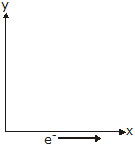I.    Velocity of the electron.
II. Magnitude of the momentum of the electron.
III. Force on the electron.
IV. The kinetic energy of electron
(A) I and II    (B) II and IV
(C) II, III and IV    (D) II and IV

1.    B    2.    C    3.    A    4.    B

5.    D    6.    C    7.    A     8.    B

9.    C    10.    D    11.    A    12.     C

13.    D    14.    B    15.    C    16.     C

17.    A    18.    C    19.     C    20.     C

21.    D    22.    C    23.    C    24.     B

25.    D    26.    D    27.    D    28.     D

29.     C    30.     D    31.     C    32.     A

33.     A    34.     B    35.     D    36.     B

37.     B    38.     A    39.     D    40.     D

41.     A    42.    D    43.     C    44.     C

45.     C    46.     B    47.    C    48.    C

49.    C    50.    D

### Objective type Questions

Choose The Correct One

1.    The fact that magnetic field is produced around a wire carrying a current, was discovered by
(1) Faraday     (2) Oersted     (3) Maxwell     (4) Joule.

2.    When the current is passing through the straight wire then, the associated magnetic field is
(1) Straight     (2) Elliptical     (3) Circular     (4) Parabolic.

3.    When current is circular, the associated magnetic field is
(1) Straight     (2) Elliptical     (3) Circular     (4) Parabolic.

4.    When current flows clockwise in a loop, the polarity of its face is
(1) East     (2) South     (3) West     (4) North.

5.    When current flows anticlockwise in a loop the magnetic polarity of the face is
(1) East     (2) South     (3) West     (4) North.

6.    For a solenoid carrying a current I and having n turns per unit length, wrapped on a core of permeability m, the correct expression for magnetic field intensity (2) is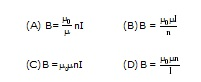7.    Magnets having temporary magnetism are called
(1) Electromagnets     (2) Bar magnets     (3) Circular magnets     (4) Horse-shoe magnets.

8.    Direction of force acting on a current carrying conductor kept in a magnetic field is given by
(1) Fleming's right hand rule     (2) Fleming's left hand rule
(3) Lenz's rule         (4) Faraday's rule.

9.    The electric device which works on the phenomenon of force on a current carrying conductor in a magnetic field is
(1) Generator     (2) Accelerator     (3) Motor     (4) Transformer.

10.    Electromagnetic induction was discovered by
(1) Oersted     (2) Maxwell.     (3) Thomson     (4) Faraday.

11.    Direction of induced current produced by motion of a conductor in a magnetic field is given by
(1) Fleming's right hand rule     (2) Fleming's left hand rule
(3) Lenz's rule         (4) Faraday's rule.

12.    In domestic electric circuits, fuse must be placed in series with
(1) Earth wire         (2) Neutral wire
(3) Live wire         (4) Any of the three wires.

13.    Electric and main switch is contained in a main board fitted usually
(1) At street electric pole         (2) At main gate of building
(3) In varandah or poarch         (4) In bed or study room.

14.    High powered electrical appliances are earthed to
(1) Avoid shock         (2) Avoid wastage
(3) Make the appliance look beautiful     (4) Reduce the bill.

15.    In a three pin socket (shoe) the bigger hole is connected to
(1) Any wire     (2) Live wire     (3) Neutral wire     (4) Earth wire.

(1) Less     (2) More     (3) Zero     (4) Not definite.

17.    Coming of live wire and neutral wire in direct contact causes

18.    Melting point of material of a fuse wire must be
(1) Moderate     (2) High     (3) Low     (4) Infinite.

19.    A high powered electric appliance has used inferior wires and is not earthed. It is a source of
(1) No concern     (2) Less concern     (3) Moderate concern     (4) Hazard.

20.    Which of the following correctly describes the magnetic field near a long straight wire?
(1) The field consists of straight lines perpendicular to the wire.
(2) The field consists of straight lines parallel to the wire.
(2) The field consists of radial lines originating from the wire.
(4) The field consists of concentric circles centred on the wire.

21.    The phenomenan of electromagnetic induction is
(1) The process of charging a body
(2) The process of generating magnetic field due to a current passing through a coil
(3) Producing induced current in a coil by relative motion between a magnet and the coil
(4) The process of rotating a coil of an electric motor.

22.    The device used for producing electric current is called a
(1) Generator     (2) Galvanometer    (3) Ammeter      (4) Motor.

23.    At the time of short-circuit, the current in the circuit
(1) Reduces substantially         (2) Does not change
(3) Increases heavily         (4) Vary continuously.

24.    An electron enters a magnetic field at right angles to it as shown in figure. The direction of force acting on the electron will be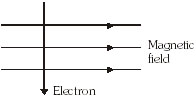(1) To the right
(2) To the left
(3) Out of the page
(4) Into the page.

25.    Which of the following property of a proton can change while it moves freely in a magnetic field? (There may be more then one correct answers)
(1) Mass     (2) Speed     (3) Velocity     (4) Momentum.

26.    A positively-charged particle (alpha particle) projected towards west is deflected towards north by a magnetic field. The direction of magnetic field is
(1) Towards south     (2) Towards east     (3) Downward     (4) Upward.

27.    A rectangular coil of copper wires is rotated in a magnetic field. The direction of the induced current changes once in each
(1) Two revolutions     (2) One revolution     (3) Half revolution     (4) One-fourth revolution.

28.    The magnetic field inside a long straight solenoid carrying current
(1) Is zero         (2) Decreases as we move towards its end
(3) Increases as we move towards its end     (4) Is the same at all points.

29.    A magnetic field exerts no force on :
(1) An electric charge moving perpendicular to its direction
(2) an unmagnetised iron bar
(3) a stationary electric charge
(4) a magnet

30.    Which of the following instruments works by electromagnetic induction ?
(1) telephone receiver         (2) simple motor
(3) dynamo         (4) moving coil galvanometer.

31.    The use of fuses in electric power-lines is :
(1) to keep main voltage constant
(2) to open the line permanently when there is an overload
(3) just to show an indication when there is an overload
(4) as a switch to use in an emergency

32.    When the main switch of the house circuit is put off, it disconnects the :
(1) live wire     (2) neutral wire     (3) earth wire     (4) live and neutral wires.

33.    When a fuse is rated at 8 A, it means :
(1) it will not work if current is less than 8 A     (2) it has a resistance of 8 ohm
(3) it will work only if current is 8 A     (4) it will burn if current exceeds 8 A.

34.    According to International Convention of colour coding of wires used in household electrical circuits :
(1) live is red, neutral is black and earth is green
(2) live is red, neutral is green and earth is black
(3) live is brown, neutral is blue and earth is black
(4) live is brown, neutral is light blue and earth is green.

35.    The magnetic field lines inside a current carrying solenoid are :
(1) along the axis and parallel to each other
(2) perpendicular to the axis and equidistant from each other
(3) circular and they do not intersect each other
(4) circular at the ends but they are parallel to the axis inside the solenoid

36.    Potential difference between a live wire and the neutral wire is :
(1) 150 V     (2) 210 V     (3) 200 V     (4) 220 V.

37.     Which of the following statements is incorrect regarding magnetic field lines :
(1)    The direction of magnetic field at a point is taken to be the direction in which the north pole of a magnetic compass needle points
(2)    Magnetic field lines are closed curves
(3)    If magnetic field lines are parallel and equidistant, they represent zero field strength
(4)    Relative strength of magnetic field is shown by the degree of closeness of the field lines

38.     Magnetic field lines determine :
(1) The shape of the magnetic field
(2) Only the direction of the magnetic field
(3) Only the relative strength of the magnetic field
(4) Both the direction and the relative strength of the magnetic field

39.     The magnetic field near a long straight wire is described by :
(1)    Straight fieldlines parallel to the wire    (2) Straight field lines perpendicular to the wire
(3)    Concentric circles centred on the wire    (4) Radial field lines starting from the wire

40.     The phenomenon of electromagnetic induction is :
(1)    The process of charging a sphere.
(2)    The process of producing magnetic field in a coil
(3)    The process of producing induced current in a coil whenever there is a relative motion between the coil and the magnet.
(4)    The process of producing cooling effect.

41.     Potential difference between a live wire and the neutral wire is :
(1) 200 volt    (2) 150 volt    (3) 210 volt    (4) 220 volt

42.    A rectangular coil of copper wires is rotated in a magnetic field. The direction of the induced current changes once in each :
(1) One revolution    (2) One-fourth revolution    (3) Half revolution    (4) Two revolutions.

43.     Magnetic field inside a long solenoid carrying current is :
(1)    Same at all points
(2)    Minimum in the middle
(3)    More at the ends than at the centre
(4)    Found to increase from one end to the other.

44.     At the time of short circuit, the current in the circuit :
(1) Vary continuously     (2) Reduces considerably    (3) Increases heavily    (4) does not change.

45.     The frequency of direct current is :
(1) Zero    (2) 50 Hz    (3) 60 Hz    (4) 100 Hz.

46.     The frequency of household supply of a.c. in India is :
(1) Zero    (2) 50 Hz    (3) 60 Hz    (4) 100 Hz.

47.     The most important safety device method used for protecting electrical appliances from short circuiting or overloading is :
(1)    Earthing    (2) Use of stabilizers    (3) Use of electric meter    (4) Use of fuse

48.     Current flowing in conductor A is 2A and current flowing in conductor B is 4A. The ratio of magnetic field produced around conductor A to the magnetic field produced around conductor B at a distance 10 cm from both the conductors is :
(1) 2 : 1    (2) 1 : 2    (3) 4 : 1    (4)  : 1

49.     Current flowing in conductors A and B is same, what is the ratio of the magnetic field produced around the conductor A at a distance of 5 cm from the conductor to the magnetic field produced around the conductor B at a distance of 2 cm from this conductor is
(1) 0.04    (2) 0.4    (3) 4.0    (4) 10

50.     Same amount of current flows in the same direction .long  the two parallel conductors separated by a small distance :
(1)    Both conductors attract each other
(2)    Both conductors repel each other
(3)    Conductors neither attract each other nor repel each other
(4)    Both conductors rotates about their axis.

51.     When an electric current flows through a long solenoid, magnetic field is set up in and around the solenoid :
(1)    Magnetic field inside the solenoid is non-uniform and weak
(2)    Magnetic field outside the solenoid is uniform and strong
(3)    Magnetic field inside the solenoid increases as we move towards the ends of the solenoid
(4)    Magnetic field of solenoid resembles the magnetic field of the bar magnet.

52.     Magnetic field produced at the centre of a current carrying circular wire is :
(1)    Directly proportional to the square of the radius of the circular wire
(2)    Directly proportional to the radius of the circular wire
(3)     Inversely proportional to the square of the radius of the circular wire
(4)    Inversely proportional to the radius of the circular wire.

53.     The direction of the magnetic field at a point P above the current carrying wire is
(1)    Down the page    (2) Up the page    (3) Into the page    (4) Out of the page.

54.     Which of the following properties of a proton can change while it moves freely in a magnetic field ?
(1) Mass    (2) Speed    (3) Velocity    (4) Momentum

55.     A positively charged particle say an alpha particle projected towards west is deflected towards north by a magnetic field. The direction of the magnetic field is :
(1)    Upward    (2) Downward    (3) Towards south    (4) Towards east

56.     Magnitude of magnetic field intensity at a point around a current carrying conductor is B. If the strength of current in the conductor becomes double, then the magnitude of magnetic field intensity at the point around the conductor is :
(1)     (2) B     (3)     (4) 2B

57.     Two parallel conductor carrying current in the same directions
(1)    Repel each other
(2)    Attract each other
(3)     Sometimes attract and sometimes repel each other
(4)    None of these

58.     Two parallel conductor carrying current in the opposite directions
(1)     Repel each other
(2)    Attract each other
(3)     Sometimes attract and sometimes repel each other
(4)    None of these

59.    Force acting on a stationary charge Q in the magnetic field B is :
(1) B Q u    (2) BQ/u    (3) Bu/Q    (4) Zero

60.     If the plug of the key is taken out (i.e. the circuit is made open) and magnetic field lines are drawn over the horizontal plane white sheet of paper, the lines are :
(1)    Concentric circles        (2) Elliptical in shape
(3)    Straight lines parallel to each other    (4) Parabolic in shape

61.     When current passes through a long straight solenoid, N and S poles are created at the two ends of the solenoid. Which of the following statemtns is incorrect ?
(1)    The field lines inside the solenoid are in the form of straight lines indicating that magnetic field is same at all the points inside the solenoid.
(2)    The pattern of the magnetic field lines associated with the solenoid is different from the pattern of the magnetic field lines around a bar magnet.
(3)    N-and S-poles exchange portions when the direction of the current through the solenoid is reversed.
(4) Magnetic field produced inside the solenoid can be used to magnetise a bar of magnetic material like soft iron, when placed inside the solenoid.
In these type of Questions, two statements are given. Choose the correct option by following directions given below : (Q. 80 to 83)
(1)    Statement I is correct and Statement II is correct explanation of the statement I.
(2)    Statement I is correct but Statement II is not the correct explanation of the statement I.
(3)    Statement I is true but statement II is not true.
(4)    Statement I is not true but statement II is true.

62.    Statement I : A soft iron bar placed inside a solenoid carrying current is magnetised.
Statemet II : Magnetic field inside a long solenoid carrying current is non-uniform.
(1) A    (2) B    (3) C    (4) D

63.    Statement I : The strength of magnetic field of a permanent magnet decreases with the increase in temperature.
Statemet II : A permanent magnet can be demagnetised by heating it.
(1) A    (2) B    (3) C    (4) D

64.    Statement I : A charged particle moving parallel to the direction of magnetic field experiences a force.
Statemet II : A charged particle moving at right angle to the direction of magnetic field experiences maximum force.
(1) A    (2) B    (3) C    (4) D

65.     A current carrying conductor placed perpendicular to the magnetic field experience a force. The displacement of this conductor in the magnetic field can be increased by.
(1)    Decreasing the magnetic field    (2) Decreasing the current in the conductor
(3)    Increasing the magnetic field    (4) Decreasing the length of the conductor.

66.     A conductor of length 50 cm, carrying current of 0.1 A, when placed perpendicular to direction of magneitc field 0.2 T experience force :
(1) 1.0 N    (2) 0.1 N    (3)0.01N    (4) 0.01 N.

67.     A charged particle having charge 3.2 × 10–19 C is travelling with a speed of 1.0 × 106 ms–1. When it passes perpendicular to the magneitc field 0.4 T, then the force experienced by it is :
(1) 12.8 × 10–13 N    (2) 1.28 × 10–13 N
(3) 19.2 × 10–15 N    (4) 1.92 × 10–15 N.

68.     A charged particle having charge 1.6 × 10–19 C travels with a speed of 3.2 × 106 ms–1 in a direction parallel to the direction of magnetic field 0.04 T. The force experienced by the particle is :
(1) 2.0 × 10–14 N    (2) 0.2 × 10–14 N
(3) Zero    (4) 4.0 × 10–14 N

69.     A magnetic compass is placed near a current carrying wire. The deflection of the needle of the magnetic compass increases. It shows that :
(1)    Current in the wire is decreasing
(2)    Current in the wire is increasing
(3)    Current in the wire has nothing to do with the deflection of the needle of the magnetic compass
(4)     Magnetic compass has been disturbed by some one.

70.     A charged particle moving in a magnetic field experience a maximum force, when it moves at right angle to the direction of magnetic field. However, a student observes that a chraged particle moving in the region of a magnetic field experiences no force and continues to move in a straight line. The observation of the student is correct only if :
(1)    Charged particle moves at an angle of 30° with the magnetic field
(2)    Charged particle moves at an angle of 60° with the magnetic field
(3)    Charged particle either moves parallel or anti-parallel to the magnetic field
(4)     Charged particle can never move in a straight line in the magnetic field.

71.    A soft iron rod can be made an electro-magnet. Which of the following procedures would be adopted to test that the soft iron rod had become a magnet ?
(1)    Place the iron rod near a bar magnet and observe if it attracts iron pins
(2)    Place the iron rod near a current carrying wire and observe if it attracts iron pins
(3)    Place the iron rod inside a currrent carrying solenoid and observe if it attacts iron pins
(4)     None of these.

72.    Current can be made to flow through a coil without connecting the coil with a battery. Which of the following procedures would be most suited to test this fact ?
(1)    Connect a galvanometer across the ends of the coil and observe if galvanometer shows a deflection, when a bar magnet is placed near the one end of the coil.
(2)    Connect a galvanometer across the ends of the coil and observe if galvanometer shows a deflection, when a bar magnet is placed inside the coil.
(3)    Connect the galvanometer across the ends of the coil and observe if galvanometer shows a deflection, when a bar magnet is moved towards or away from the coil and along the axis of the coil.
(4)     Connect the galvanometer across the ends of the coil and observe if galvanometer shows a deflection, when a bar magnet is moved at right to the axis of the coil.

73.     Two coil A and B placed close to each other. If current in the coil A is changed, some current will be induced in the coil B. This is because of electromagnetic induction. In this statement :
(1)    Inference is correct but reasoning is not correct
(2)     Inference is incorrect but reasong is correct
(3)    Inference as well as reasoning are correct
(4)     Neither inference nor reasoning is correct.